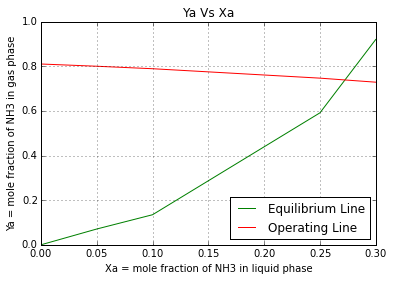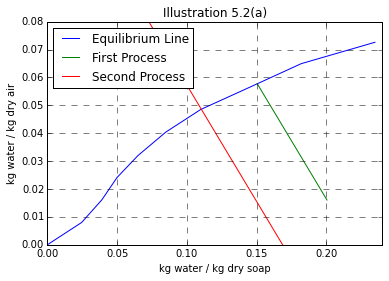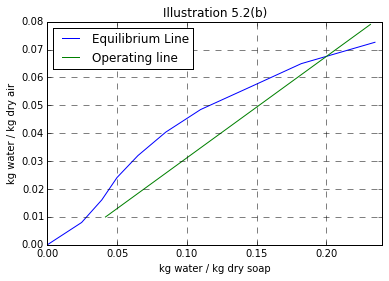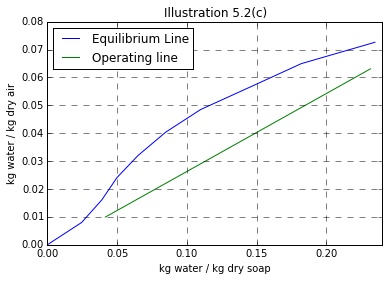Chapter 5: Interphase Mass Transfer¶

Ex5.1: Page 114¶

In :
# Illustration 5.1
# Page: 114

print'Illustration 5.1 - Page: 114\n\n'
import matplotlib.pyplot as plt
import numpy
%matplotlib inline
# solution

#***Data***#
# a = NH3, b = H2O
d = 2.54*10**(-2);# [m]
Yag = 0.80;
Xal = 0.05;
T = 273+26.7;# [K]
Kl = 2.87*10**(-5);# [kmol/square m.s.(kmol/cubic m)]
Sh = 40;
Da = 2.297*10**(-5);# [square m.s]
P = 1.0133*10**(5);# [N/square m]
Xbm = 1.0;
#*********#

Ma = 18.0;# [kg/kmol]
# Liquid:
# Because of large conc. of ammonia in gas F's rather than k's are used.
# Molecular weight of water and ammonia are nearly same.
# The density of the solution is practically that of water.
MolarDensity1 = 1000/Ma;# [kmol/cubic m]
# Kl is determined for dilute soln. where Xbm is practically 1.0
Fl = Kl*Xbm*MolarDensity1;# [kmol/square m.s]
Ma = 18;# [kg-/kmol]
# Gas:
MolarDensity2 = (1/22.41)*(273/(273+26.7));# [kmol/cubic m]
Fg = Sh*MolarDensity2*Da/d;# [kmol/square m.s]

# Mass Transfer Flux
# Th eqb. distribuion data for NH3 from "The Chemical Engineers Handbook" 5th Edt. p3-68:
# Data = [Xa,pa]
# Xa = NH3 mole fraction in gas phas
# pa = NH3 partial pressure in N/square m
Data = [(0 ,0),(0.05 ,7171),(0.10, 13652),(0.25 ,59917),(0.30 ,93220)];

X = numpy.zeros(5);
for i in range(1,5) :
X[i]=Data[i]

# Ya_star = mole fraction of NH3 in gas phase at eqb.
Ya_star = numpy.zeros(5);
for i in range(0,5) :
Ya_star[i] = (Data[i])/P

# For transfer of only one component
Na_by_SummationN = 1.0;
Ya = numpy.zeros(5);
for i in range(0,5):
Ya[i] = 1-((1-Yag)*(1.0-Xal)/(1-Data[i]));

plt.plot(X,Ya_star,'g',label='Equilibrium Line')
plt.plot(X,Ya,'r',label='Operating Line')
ax = pylab.gca()
ax.grid('on')
ax.set_xlabel('Xa = mole fraction of NH3 in liquid phase');
ax.set_ylabel('Ya = mole fraction of NH3 in gas phase');
pylab.legend(loc='lower right')
plt.title('Ya Vs Xa');
plt.show()

# From intersection of operating line & Eqb. line
Xai = 0.274;
Yai = 0.732;

# From Eqn.5.20
Na = Na_by_SummationN*Fg*log((Na_by_SummationN-Yai)/(Na_by_SummationN-Yag));# [kmol NH3 absorbed/square m.s]
print"Local mass transfer flux for ammonia is ",round(Na,6)," kmol/square m.s"
Illustration 5.1 - Page: 114Local mass transfer flux for ammonia is  0.00043  kmol/square m.s

Ex5.2: Page 130¶

In :
# Illustration 5.2
# Page: 130

import matplotlib.pyplot as plt
%matplotlib inline
import numpy

print'Illustration 5.2 - Page: 130\n\n'

# solution

#****Data***#
# Eqb. data
# Data = [Wt% of moisture in the soap,Partial pressure of water in air(mm Hg)]
Data = [(0,0),( 2.40, 9.66),(3.76 ,19.20),(4.76 ,28.4),(6.10, 37.2),(7.83, 46.4),(9.90, 55.0),(12.63, 63.2),(15.40, 71.9),(19.02 ,79.5)];
P = 760.0;# [mm Hg]
# Initial air
p1 = 12;# [mm Hg]
T = 273+75.0;# [K]
#******#

# Y = kg water/kg dry air
# X = kg water/kg dry soap
# E = Air water phase
# R = Soap water phase
Y = numpy.zeros(10);
X = numpy.zeros(10);
for i in range(1,10):
Y[i] = Data[i]/(P-Data[i])*(18.02/29);
X[i] = Data[i]/(100.0-Data[i]);

print'Illustration 5.2 (a)\n\n'

import pylab
# Soln. (a)
# First operation
Y1 = p1/(P-p1);# [kg water/kg dry soap]
# Initial Soap
S1 = 16.7/(100-16.7);# [kg water/kg dry soap]
# Final soap
S2 = 13.0/(100-13);# [kg water/kg dry soap]
Rs = 10.0*(1-0.167);# [kg dry soap]
# Using ideal gas law
Es = 10.0*((760-p1)/760.0)*(273.0/T)*(29.0/22.41);# [kg dry air]
slopeOperat = -Rs/Es;

def f2(x):
return slopeOperat*(x-S1)+Y1
x = numpy.arange(S1,S2,-0.01);
X1=S2;
def f3(S):
return slopeOperat*(S-X1)+Y1
S=numpy.arange(0,S1,0.01);

plt.plot(X,Y,'blue',label='Equilibrium Line')
plt.plot(x,f2(x),'g',label='First Process')
plt.plot(S,f3(S),'r',label='Second Process')
ax = pylab.gca()
plt.title("Illustration 5.2(a)")
ax.set_autoscale_on('False')
pylab.axis([0.0,0.24, 0,0.08])
plt.grid(b=None, which='major', axis='both')
ax.grid(color='Black', linestyle='--', linewidth=0.5)
pylab.legend(loc='upper left')
ax.set_xlabel('kg water / kg dry soap')
ax.set_ylabel('kg water / kg dry air')
plt.show()

# Results for First Process
# The condition at abcissa S2 correspond to the end of first operation
print "Conditions corresponding to First Operation \n"
print "X =  kg water/kg dry soap\n",S2
print "Y =  kg water/kg dry air\n",f2(S2)

# Results for Second Process
#  The point at which the line meets the equilibrium line corresponds to the final value
X2 = 0.103;
Y2 = (X2/(1+X2));
print"Final moisture content of soap is ",round(Y2*100,3),'%'

print'\n\n Illustration 5.2 (b)\n\n'

# Solution (b)

Rs = 1*(1-0.167);# [kg dry soap/h]
# Entering soap
X1 = 0.20;# [kg water/kg dry soap]
# Leaving soap
x = 0.04;
X2 = x/(1-x);# [kg water/kg dry soap]
# Entering air
Y2 = 0.00996;# [from Illustration 5.2(a), kg water/kg dry air]
# The operating line of least slope giving rise to eqb. condition will indicate least amount of air usable.
# At X1 = 0.20; the eqb. condition:
Y1 = 0.0675;# [kg water/kg dry air]

def f4(x):
return ((Y1-Y2)/(X1-X2))*(x-X1)+Y1
x = numpy.arange(X2,0.24,0.01);
plt.plot(X,Y,'blue',label='Equilibrium Line')
plt.plot(x,f4(x),'g',label='Operating line')
ax = pylab.gca()
ax.set_xlabel('kg water / kg dry soap')
ax.set_ylabel('kg water / kg dry air')
ax.set_autoscale_on('False')
pylab.axis([0.0,0.24, 0,0.08])
plt.title("Illustration 5.2(b)")
plt.grid(b=None, which='major', axis='both')
ax.grid(color='Black', linestyle='--', linewidth=0.5)
pylab.legend(loc='upper left')
plt.show()
# By Eqn. 5.35

Es = Rs*(X1-X2)/(Y1-Y2);# [kg dry air/h]
Esv = (Es/29)*22.41*(P/(P-p1))*(T/273.0); #[cubic m/kg dry soap]
print"Minimum amount of air required is",round(Esv,4)," cubic m/kg dry soap\n\n"

print'Illustration 5.2 (c)\n\n'

# solution (c)

Esnew = 1.30*Es;# [kg dry air/h]
Y1 = Rs*((X1-X2)/Esnew)+Y2;

def f5(x):
return ((Y1-Y2)/(X1-X2))*(x-X1)+Y1
x = numpy.arange(X2,0.24,0.01);
plt.plot(X,Y,'blue',label='Equilibrium Line')
plt.plot(x,f5(x),'g',label='Operating line')
ax = pylab.gca()
ax.set_xlabel('kg water / kg dry soap')
ax.set_ylabel('kg water / kg dry air')
ax.set_autoscale_on('False')
pylab.axis([0.0,0.24, 0,0.08])
plt.title("Illustration 5.2(c)")
plt.grid(b=None, which='major', axis='both')
ax.grid(color='Black', linestyle='--', linewidth=0.5)
pylab.legend(loc='upper left')
plt.show()
# with final coordinates X = X1 & y = Y1
# From figure, Total number of eqb . stages = 3
N = 3;
print"Moisture content of air leaving the drier is ",round(Y1,4)," kg water/kg dry air\n"
print"Total number of eqb. stages = ",N
Illustration 5.2 - Page: 130

Illustration 5.2 (a)Conditions corresponding to First Operation

X =  kg water/kg dry soap
0.149425287356
Y =  kg water/kg dry air
0.0586080045715
Final moisture content of soap is  9.338 %

Illustration 5.2 (b)Minimum amount of air required is 2.2941  cubic m/kg dry soap

Illustration 5.2 (c)Moisture content of air leaving the drier is  0.0542  kg water/kg dry air

Total number of eqb. stages =  3